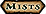Returns a random number within the specified interval.

```rand = fastrandom([ [low, ] high])
```

## Arguments

low
number - lower integer limit on the returned random value.
high
number - upper integer limit on the returned random value.

## Returns

rand
number - Generated random value. If no arguments were specified, the value is a uniformly-distributed decimal between 0 (inclusive) and 1 (exclusive). Otherwise, returns a uniformly-distributed integer between `low` (1 if not specified) and `high`, both bounds inclusive.

## Details

• This function uses C's `rand` function as a random number generator. On the Windows client, the implementation of `random(high)` is equivalent to the following, which is not cryptographically secure: it is possible to derive its internal state from a few samples of its output and correctly predict the results of subsequent calls.
```local state = 0 -- initialized by seeding
function random(high)
state = (state * 214013 + 2531011) % 2^32;
local rand = math.floor(state / 2^16) % 2^15;
return 1 + math.floor(rand / 0x7fff * high)
end
```
• Lua's `math.randomseed` is not available in World of Warcraft; the random number generator is instead seeded by the client at startup.Patch 5.4.2 / API (2013-12-10): Name changed to `fastrandom`; random and `math.random` now use a different RNG algorithm.